# Calculation of some physical quantities in the orbital path of an artificial satellite

in StemSocial5 months ago (edited)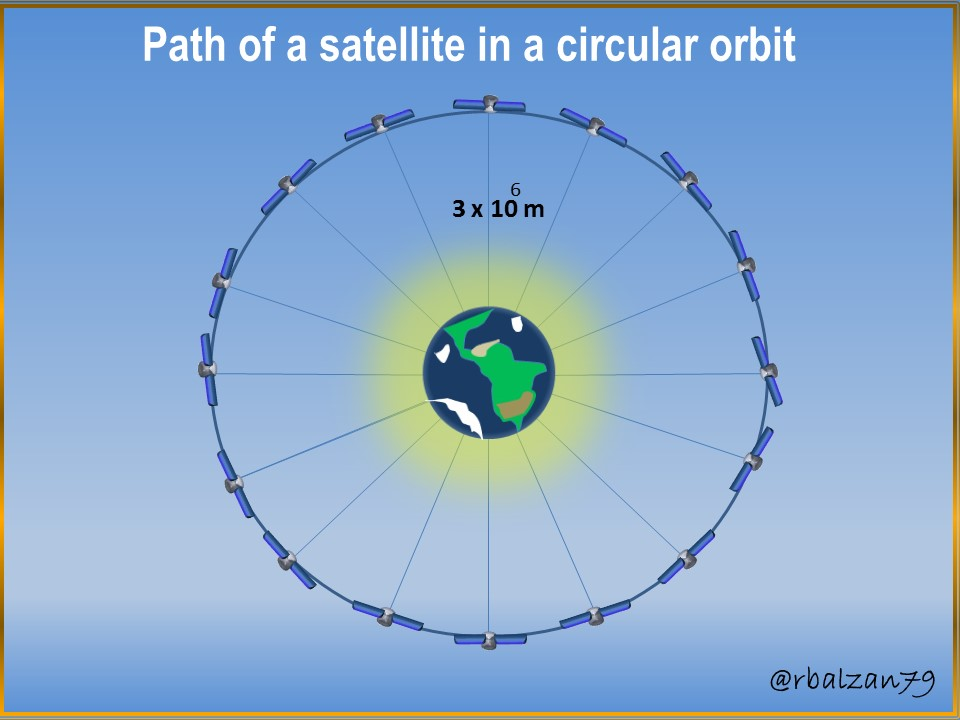Greetings again my dear friends I hope you are all well, our creativity has led us to the configuration of any type of machine or instrument which have facilitated our complex existence, of course, all this as a result of the wonderful structuring of the field of science-technology, which is why we must never fail to highlight the enormous work of these fields mentioned above, as they have been extremely fruitful areas for our development in all possible directions.

If there are some devices that have made us take an exponential leap in relation to our evolution, are artificial satellites, any person on the planet easily to speak of a satellite immediately relate it to important activities such as mass communication, for example, telephones, television and especially with the internet, many have been the purposes for its creation, as essential areas of our development implement it as education, science itself, among many other areas.

Although from the earth's crust we cannot observe them with the naked eye, we are witnesses of their great impact and influence on humanity, a natural or artificial satellite orbits around another body of greater dimension or size, that is why, in this opportunity I wanted to relate to the calculation of some physical magnitudes related to the movement of these artificial satellite equipment, therefore, in relation to these characteristics it is important to know and develop the following statement.

Exercise

A large number of artificial satellites orbit around the planet Earth, therefore, it is necessary to know some characteristics of one of these satellites, whose movement is carried out within the geometric space of a circular orbit, that is why, in relation to the above, to answer the following questions:

a.- What will be the velocity of such a satellite with circular orbit which is at a distance of 3000 km from the Earth?

b.- What is the time it takes for such a satellite to go around its orbit around our planet?

Data: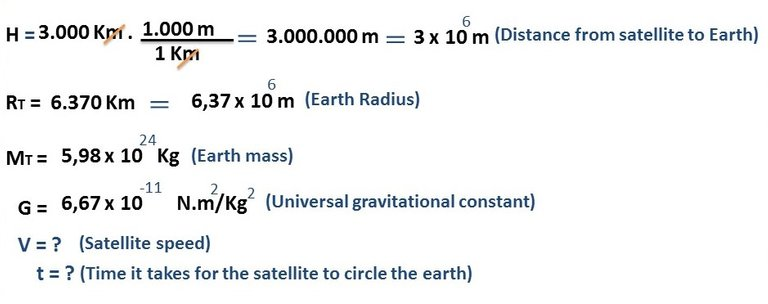Solution

a.- To begin to answer our first question, it is important to point out that since this is a circular motion, we will have to consider the action of centripetal acceleration, since the force responsible for attracting the satellite is the gravitational force of the larger body on which the satellite rotates, in this case, the Earth: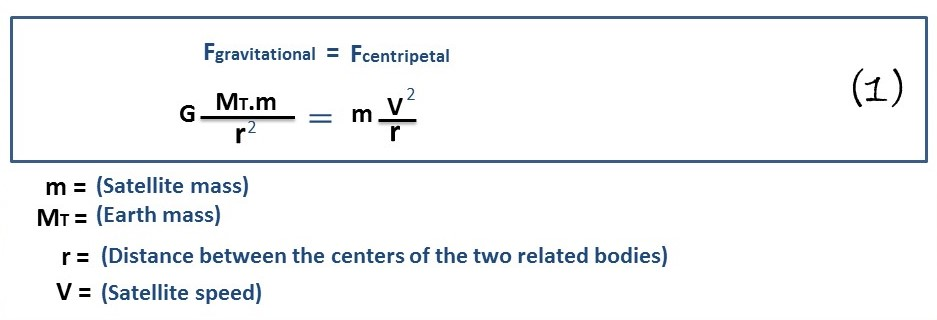Once both formulations are related, in relation to the forces linked to the circular motion that describes the orbit of our satellite to be analyzed, we proceed to simplify this equation in order to find the requested variable, in this case, the satellite velocity (V), therefore, we proceed as follows: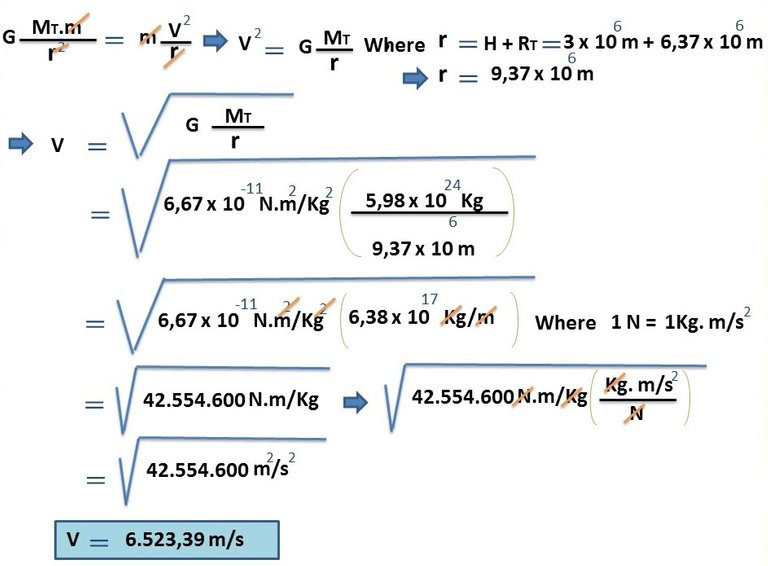In this way we obtain the velocity of the satellite moving in a circular orbit at a distance of 3,000 km above the Earth, once this important variable of velocity (V) is known, we can then move on to the following question.

b.- To begin to answer this question, it is necessary to say that since it is a circular orbital trajectory, then, the distance included in one turn will be its perimeter and we can determine it with 2π.r, also considering that it is a uniform circular motion, the velocity will be constant throughout the circular orbit, therefore, we can apply the following equation.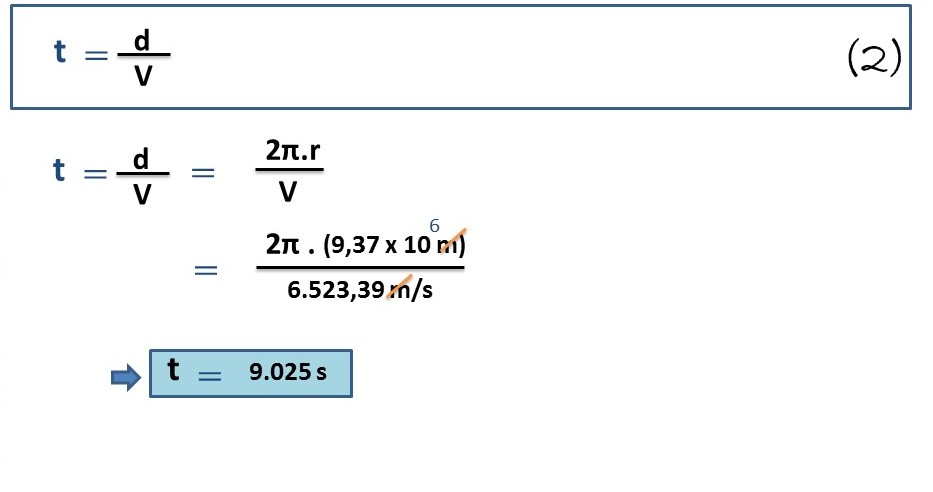In this way we were able to find the time (in seconds) that the satellite will take to travel its circular orbit around the Earth, which would represent 2.51 hours approximately (9025 s/3600s) or 2 hours 30 minutes and 36 seconds approximately, in this way we were able to know and give answers to the two questions posed above with the help of important mathematical formulations established by the essential field of science.

# Conclusion

How many forms of learning we obtain through the different formulations established by years of experimentation and analysis, in this way in a few hours or minutes we can understand the development of any type of phenomenon that develops around us, as in this case the movement through a circular orbit of a certain artificial satellite at an altitude of 3,000 km above our planet Earth.

We proposed to know some physical magnitudes of the circular orbital motion of an artificial satellite with the height described above and also knowing other important variables already established as the radius of the Earth (RT), its mass (MT) and the universal gravitational constant (G), in this way we were able to calculate both the speed of the satellite and the time it takes the same (satellite) to make a turn in its circular orbit around the Earth.

The purpose is to continue applying any type of formulation that can lead us to the understanding of any kind of phenomenon developed by any type of invention or creation of man, such as artificial satellites, which are of enormous importance in our lives.

Until another opportunity my dear friends.

Note: The images were created by the author using Power Point, the animated gif was created using the PhotoScape application.

# Recommended Bibliographic Reference